# 12.5 Conic sections in polar coordinates  (Page 6/8)

 Page 6 / 8

$\frac{{x}^{2}}{9}-\frac{{y}^{2}}{16}=1$

$\frac{{\left(y-1\right)}^{2}}{49}-\frac{{\left(x+1\right)}^{2}}{4}=1$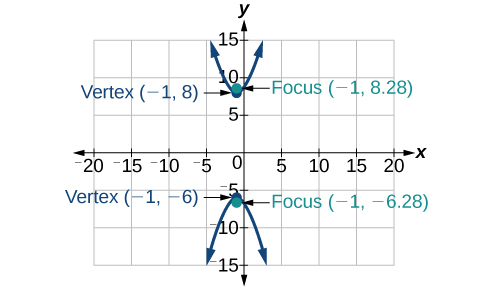${x}^{2}-4{y}^{2}+6x+32y-91=0$

$2{y}^{2}-{x}^{2}-12y-6=0$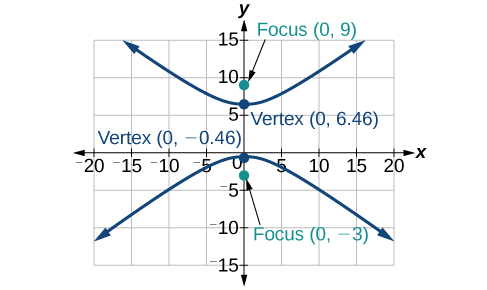For the following exercises, find the equation of the hyperbola.

Center at $\text{\hspace{0.17em}}\left(0,0\right),$ vertex at $\text{\hspace{0.17em}}\left(0,4\right),$ focus at $\text{\hspace{0.17em}}\left(0,-6\right)$

Foci at $\text{\hspace{0.17em}}\left(3,7\right)\text{\hspace{0.17em}}$ and $\text{\hspace{0.17em}}\left(7,7\right),$ vertex at $\text{\hspace{0.17em}}\left(6,7\right)$

$\frac{{\left(x-5\right)}^{2}}{1}-\frac{{\left(y-7\right)}^{2}}{3}=1$

## The Parabola

For the following exercises, write the equation of the parabola in standard form. Then give the vertex, focus, and directrix.

${y}^{2}=12x$

${\left(x+2\right)}^{2}=\frac{1}{2}\left(y-1\right)$

${\left(x+2\right)}^{2}=\frac{1}{2}\left(y-1\right);\text{\hspace{0.17em}}$ vertex: $\text{\hspace{0.17em}}\left(-2,1\right);\text{\hspace{0.17em}}$ focus: $\text{\hspace{0.17em}}\left(-2,\frac{9}{8}\right);\text{\hspace{0.17em}}$ directrix: $\text{\hspace{0.17em}}y=\frac{7}{8}$

${y}^{2}-6y-6x-3=0$

${x}^{2}+10x-y+23=0$

${\left(x+5\right)}^{2}=\left(y+2\right);\text{\hspace{0.17em}}$ vertex: $\text{\hspace{0.17em}}\left(-5,-2\right);\text{\hspace{0.17em}}$ focus: $\text{\hspace{0.17em}}\left(-5,-\frac{7}{4}\right);\text{\hspace{0.17em}}$ directrix: $\text{\hspace{0.17em}}y=-\frac{9}{4}$

For the following exercises, graph the parabola, labeling vertex, focus, and directrix.

${x}^{2}+4y=0$

${\left(y-1\right)}^{2}=\frac{1}{2}\left(x+3\right)$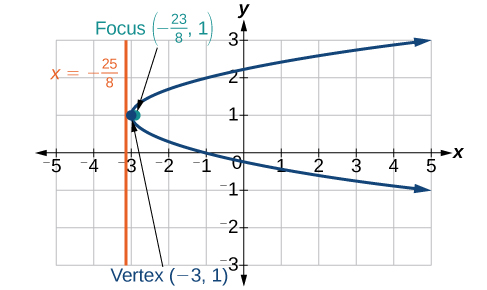${x}^{2}-8x-10y+46=0$

$2{y}^{2}+12y+6x+15=0$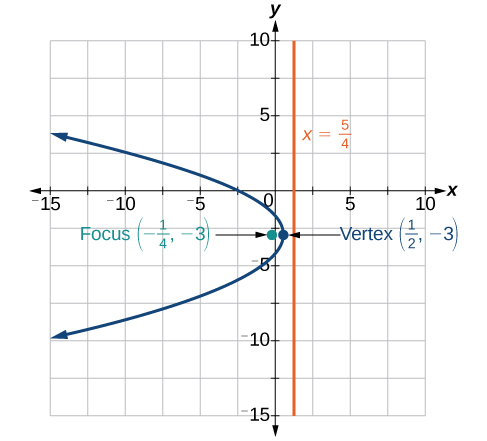For the following exercises, write the equation of the parabola using the given information.

Focus at $\text{\hspace{0.17em}}\left(-4,0\right);\text{\hspace{0.17em}}$ directrix is $\text{\hspace{0.17em}}x=4$

Focus at $\text{\hspace{0.17em}}\left(2,\frac{9}{8}\right);\text{\hspace{0.17em}}$ directrix is $\text{\hspace{0.17em}}y=\frac{7}{8}$

${\left(x-2\right)}^{2}=\left(\frac{1}{2}\right)\left(y-1\right)$

A cable TV receiving dish is the shape of a paraboloid of revolution. Find the location of the receiver, which is placed at the focus, if the dish is 5 feet across at its opening and 1.5 feet deep.

## Rotation of Axes

For the following exercises, determine which of the conic sections is represented.

$16{x}^{2}+24xy+9{y}^{2}+24x-60y-60=0$

${B}^{2}-4AC=0,$ parabola

$4{x}^{2}+14xy+5{y}^{2}+18x-6y+30=0$

$4{x}^{2}+xy+2{y}^{2}+8x-26y+9=0$

${B}^{2}-4AC=-31<0,$ ellipse

For the following exercises, determine the angle $\text{\hspace{0.17em}}\theta \text{\hspace{0.17em}}$ that will eliminate the $\text{\hspace{0.17em}}xy\text{\hspace{0.17em}}$ term, and write the corresponding equation without the $\text{\hspace{0.17em}}xy\text{\hspace{0.17em}}$ term.

${x}^{2}+4xy-2{y}^{2}-6=0$

${x}^{2}-xy+{y}^{2}-6=0$

$\theta ={45}^{\circ },{{x}^{\prime }}^{2}+3{{y}^{\prime }}^{2}-12=0$

For the following exercises, graph the equation relative to the $\text{\hspace{0.17em}}{x}^{\prime }{y}^{\prime }\text{\hspace{0.17em}}$ system in which the equation has no $\text{\hspace{0.17em}}{x}^{\prime }{y}^{\prime }\text{\hspace{0.17em}}$ term.

$9{x}^{2}-24xy+16{y}^{2}-80x-60y+100=0$

${x}^{2}-xy+{y}^{2}-2=0$

$\theta ={45}^{\circ }$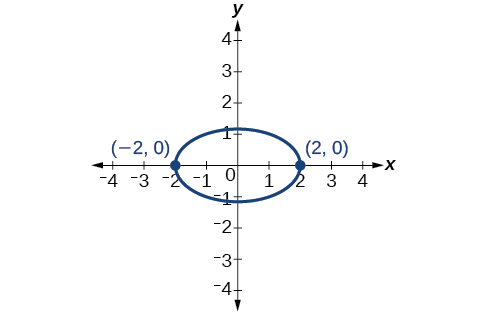$6{x}^{2}+24xy-{y}^{2}-12x+26y+11=0$

## Conic Sections in Polar Coordinates

For the following exercises, given the polar equation of the conic with focus at the origin, identify the eccentricity and directrix.

Hyperbola with $\text{\hspace{0.17em}}e=5\text{\hspace{0.17em}}$ and directrix $\text{\hspace{0.17em}}2\text{\hspace{0.17em}}$ units to the left of the pole.

Ellipse with $\text{\hspace{0.17em}}e=\frac{3}{4}\text{\hspace{0.17em}}$ and directrix $\text{\hspace{0.17em}}\frac{1}{3}\text{\hspace{0.17em}}$ unit above the pole.

For the following exercises, graph the conic given in polar form. If it is a parabola, label the vertex, focus, and directrix. If it is an ellipse or a hyperbola, label the vertices and foci.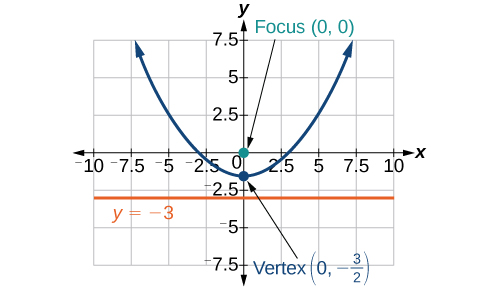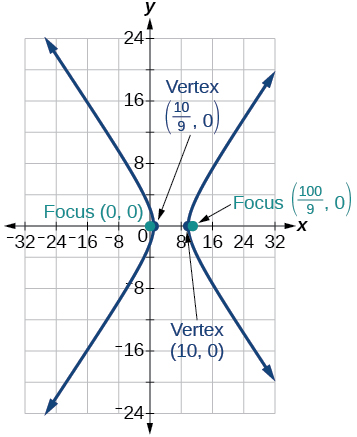For the following exercises, given information about the graph of a conic with focus at the origin, find the equation in polar form.

Directrix is $\text{\hspace{0.17em}}x=3\text{\hspace{0.17em}}$ and eccentricity $\text{\hspace{0.17em}}e=1$

Directrix is $\text{\hspace{0.17em}}y=-2\text{\hspace{0.17em}}$ and eccentricity $\text{\hspace{0.17em}}e=4$

## Practice test

For the following exercises, write the equation in standard form and state the center, vertices, and foci.

$\frac{{x}^{2}}{9}+\frac{{y}^{2}}{4}=1$

$\frac{{x}^{2}}{{3}^{2}}+\frac{{y}^{2}}{{2}^{2}}=1;\text{\hspace{0.17em}}$ center: $\text{\hspace{0.17em}}\left(0,0\right);\text{\hspace{0.17em}}$ vertices: $\text{\hspace{0.17em}}\left(3,0\right),\left(–3,0\right),\left(0,2\right),\left(0,-2\right);\text{\hspace{0.17em}}$ foci: $\left(\sqrt{5},0\right),\left(-\sqrt{5},0\right)$

$9{y}^{2}+16{x}^{2}-36y+32x-92=0$

For the following exercises, sketch the graph, identifying the center, vertices, and foci.

$\frac{{\left(x-3\right)}^{2}}{64}+\frac{{\left(y-2\right)}^{2}}{36}=1$

center: $\text{\hspace{0.17em}}\left(3,2\right);\text{\hspace{0.17em}}$ vertices: $\text{\hspace{0.17em}}\left(11,2\right),\left(-5,2\right),\left(3,8\right),\left(3,-4\right);\text{\hspace{0.17em}}$ foci: $\text{\hspace{0.17em}}\left(3+2\sqrt{7},2\right),\left(3-2\sqrt{7},2\right)$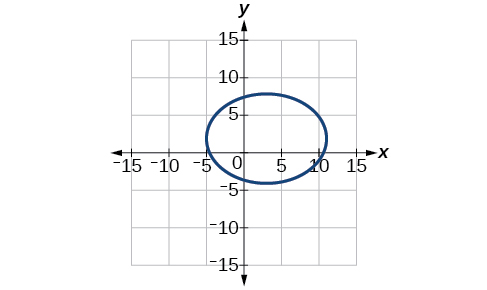$2{x}^{2}+{y}^{2}+8x-6y-7=0$

Write the standard form equation of an ellipse with a center at $\text{\hspace{0.17em}}\left(1,2\right),$ vertex at $\text{\hspace{0.17em}}\left(7,2\right),$ and focus at $\text{\hspace{0.17em}}\left(4,2\right).$

$\frac{{\left(x-1\right)}^{2}}{36}+\frac{{\left(y-2\right)}^{2}}{27}=1$

A whispering gallery is to be constructed with a length of 150 feet. If the foci are to be located 20 feet away from the wall, how high should the ceiling be?

For the following exercises, write the equation of the hyperbola in standard form, and give the center, vertices, foci, and asymptotes.

$\frac{{x}^{2}}{49}-\frac{{y}^{2}}{81}=1$

$\frac{{x}^{2}}{{7}^{2}}-\frac{{y}^{2}}{{9}^{2}}=1;\text{\hspace{0.17em}}$ center: $\text{\hspace{0.17em}}\left(0,0\right);\text{\hspace{0.17em}}$ vertices $\text{\hspace{0.17em}}\left(7,0\right),\left(-7,0\right);\text{\hspace{0.17em}}$ foci: $\text{\hspace{0.17em}}\left(\sqrt{130},0\right),\left(-\sqrt{130},0\right);\text{\hspace{0.17em}}$ asymptotes: $\text{\hspace{0.17em}}y=±\frac{9}{7}x$

$16{y}^{2}-9{x}^{2}+128y+112=0$

For the following exercises, graph the hyperbola, noting its center, vertices, and foci. State the equations of the asymptotes.

$\frac{{\left(x-3\right)}^{2}}{25}-\frac{{\left(y+3\right)}^{2}}{1}=1$

center: $\text{\hspace{0.17em}}\left(3,-3\right);\text{\hspace{0.17em}}$ vertices: $\text{\hspace{0.17em}}\left(8,-3\right),\left(-2,-3\right);$ foci: $\text{\hspace{0.17em}}\left(3+\sqrt{26},-3\right),\left(3-\sqrt{26},-3\right);\text{\hspace{0.17em}}$ asymptotes: $\text{\hspace{0.17em}}y=±\frac{1}{5}\left(x-3\right)-3$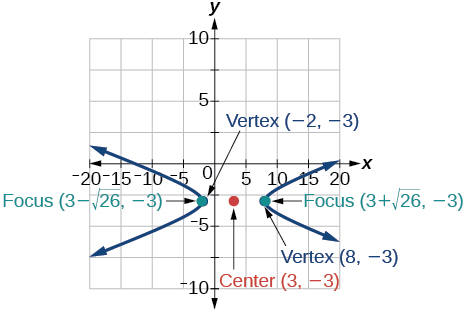${y}^{2}-{x}^{2}+4y-4x-18=0$

Write the standard form equation of a hyperbola with foci at $\text{\hspace{0.17em}}\left(1,0\right)\text{\hspace{0.17em}}$ and $\text{\hspace{0.17em}}\left(1,6\right),$ and a vertex at $\text{\hspace{0.17em}}\left(1,2\right).$

$\frac{{\left(y-3\right)}^{2}}{1}-\frac{{\left(x-1\right)}^{2}}{8}=1$

For the following exercises, write the equation of the parabola in standard form, and give the vertex, focus, and equation of the directrix.

${y}^{2}+10x=0$

$3{x}^{2}-12x-y+11=0$

${\left(x-2\right)}^{2}=\frac{1}{3}\left(y+1\right);\text{\hspace{0.17em}}$ vertex: $\text{\hspace{0.17em}}\left(2,-1\right);\text{\hspace{0.17em}}$ focus: $\text{\hspace{0.17em}}\left(2,-\frac{11}{12}\right);\text{\hspace{0.17em}}$ directrix: $\text{\hspace{0.17em}}y=-\frac{13}{12}$

For the following exercises, graph the parabola, labeling the vertex, focus, and directrix.

${\left(x-1\right)}^{2}=-4\left(y+3\right)$

${y}^{2}+8x-8y+40=0$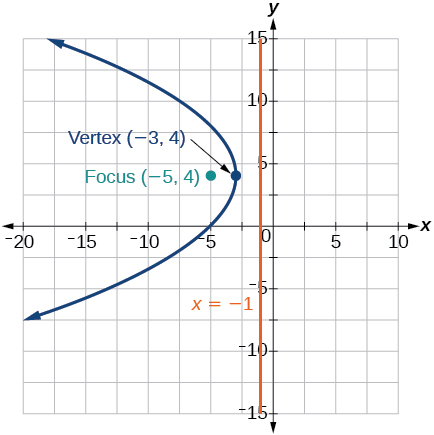Write the equation of a parabola with a focus at $\text{\hspace{0.17em}}\left(2,3\right)\text{\hspace{0.17em}}$ and directrix $\text{\hspace{0.17em}}y=-1.$

A searchlight is shaped like a paraboloid of revolution. If the light source is located 1.5 feet from the base along the axis of symmetry, and the depth of the searchlight is 3 feet, what should the width of the opening be?

Approximately $\text{\hspace{0.17em}}8.49\text{\hspace{0.17em}}$ feet

For the following exercises, determine which conic section is represented by the given equation, and then determine the angle $\text{\hspace{0.17em}}\theta \text{\hspace{0.17em}}$ that will eliminate the $\text{\hspace{0.17em}}xy\text{\hspace{0.17em}}$ term.

$3{x}^{2}-2xy+3{y}^{2}=4$

${x}^{2}+4xy+4{y}^{2}+6x-8y=0$

parabola; $\text{\hspace{0.17em}}\theta \approx {63.4}^{\circ }$

For the following exercises, rewrite in the $\text{\hspace{0.17em}}{x}^{\prime }{y}^{\prime }\text{\hspace{0.17em}}$ system without the $\text{\hspace{0.17em}}{x}^{\prime }{y}^{\prime }\text{\hspace{0.17em}}$ term, and graph the rotated graph.

$11{x}^{2}+10\sqrt{3}xy+{y}^{2}=4$

$16{x}^{2}+24xy+9{y}^{2}-125x=0$

${{x}^{\prime }}^{2}-4{x}^{\prime }+3{y}^{\prime }=0$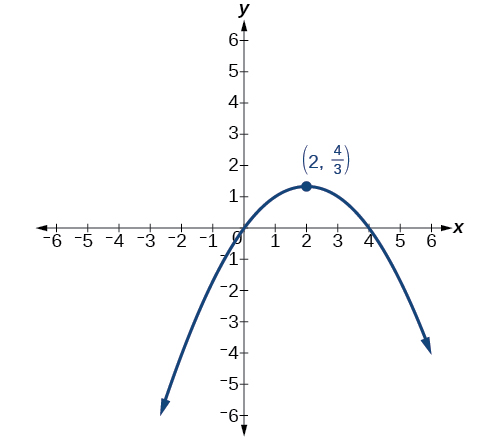For the following exercises, identify the conic with focus at the origin, and then give the directrix and eccentricity.

Hyperbola with $\text{\hspace{0.17em}}e=\frac{3}{2},\text{\hspace{0.17em}}$ and directrix $\text{\hspace{0.17em}}\frac{5}{6}\text{\hspace{0.17em}}$ units to the right of the pole.

For the following exercises, graph the given conic section. If it is a parabola, label vertex, focus, and directrix. If it is an ellipse or a hyperbola, label vertices and foci.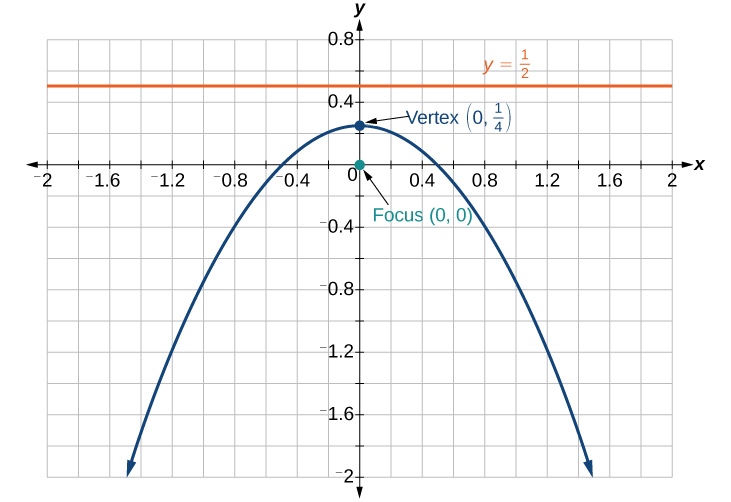Find a polar equation of the conic with focus at the origin, eccentricity of $\text{\hspace{0.17em}}e=2,$ and directrix: $\text{\hspace{0.17em}}x=3.$

sinx sin2x is linearly dependent
what is a reciprocal
The reciprocal of a number is 1 divided by a number. eg the reciprocal of 10 is 1/10 which is 0.1
Shemmy
Reciprocal is a pair of numbers that, when multiplied together, equal to 1. Example; the reciprocal of 3 is ⅓, because 3 multiplied by ⅓ is equal to 1
Jeza
each term in a sequence below is five times the previous term what is the eighth term in the sequence
I don't understand how radicals works pls
How look for the general solution of a trig function
stock therom F=(x2+y2) i-2xy J jaha x=a y=o y=b
sinx sin2x is linearly dependent
cr
root under 3-root under 2 by 5 y square
The sum of the first n terms of a certain series is 2^n-1, Show that , this series is Geometric and Find the formula of the n^th
cosA\1+sinA=secA-tanA
Wrong question
why two x + seven is equal to nineteen.
The numbers cannot be combined with the x
Othman
2x + 7 =19
humberto
2x +7=19. 2x=19 - 7 2x=12 x=6
Yvonne
because x is 6
SAIDI
what is the best practice that will address the issue on this topic? anyone who can help me. i'm working on my action research.
simplify each radical by removing as many factors as possible (a) √75
how is infinity bidder from undefined?
what is the value of x in 4x-2+3
give the complete question
Shanky
4x=3-2 4x=1 x=1+4 x=5 5x
Olaiya
hi can you give another equation I'd like to solve it
Daniel
what is the value of x in 4x-2+3
Olaiya
if 4x-2+3 = 0 then 4x = 2-3 4x = -1 x = -(1÷4) is the answer.
Jacob
4x-2+3 4x=-3+2 4×=-1 4×/4=-1/4
LUTHO
then x=-1/4
LUTHO
4x-2+3 4x=-3+2 4x=-1 4x÷4=-1÷4 x=-1÷4
LUTHO
A research student is working with a culture of bacteria that doubles in size every twenty minutes. The initial population count was  1350  bacteria. Rounding to five significant digits, write an exponential equation representing this situation. To the nearest whole number, what is the population size after  3  hours?
f(x)= 1350. 2^(t/20); where t is in hours.
MerkebByByBy Kimberly NicholsBy OpenStaxBy Bonnie HurstBy OpenStaxBy Brooke DelaneyBy Charles JumperBy Anonymous UserBy Madison ChristianBy CB BiernBy Yacoub Jayoghli